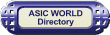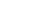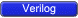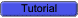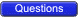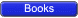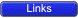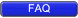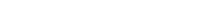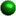Hierarchical Identifiers

Hierarchical path names are based on the top module identifier followed by module instant identifiers, separated by periods.This is useful basically when we want to see the signal inside a lower module, or want to force a value inside an internal module. The example below shows how to monitor the value of an internal module signal.Example```
1 //-----------------------------------------------------
2 // This is simple adder Program
3 // Design Name : adder_hier
4 // File Name   : adder_hier.v
5 // Function    : This program shows verilog hier path works
6 // Coder       : Deepak
7 //-----------------------------------------------------
10 result        , // Output of the adder
11 carry         , // Carry output of adder
12 r1            , // first input
13 r2            , // second input
14 ci              // carry input
15 );
16
17 // Input Port Declarations
18 input    [3:0]   r1         ;
19 input    [3:0]   r2         ;
20 input            ci         ;
21
22 // Output Port Declarations
23 output   [3:0]  result      ;
24 output          carry       ;
25
26 // Port Wires
27 wire     [3:0]    r1        ;
28 wire     [3:0]    r2        ;
29 wire              ci        ;
30 wire     [3:0]    result    ;
31 wire              carry     ;
32
33 // Internal variables
34 wire              c1        ;
35 wire              c2        ;
36 wire              c3        ;
37
38 // Code Starts Here
43
44 endmodule // End Of Module adder
45
46 module tb();
47
48 reg [3:0] r1,r2;
49 reg  ci;
50 wire [3:0] result;
51 wire  carry;
52
53 // Drive the inputs
54 initial begin
55   r1 = 0;
56   r2 = 0;
57   ci = 0;
58    #10  r1 = 10;
59    #10  r2 = 2;
60    #10  ci = 1;
61    #10  \$display("+--------------------------------------------------------+");
62   \$finish;
63 end
64
65 // Connect the lower module
67
68 // Hier demo here
69 initial begin
70   \$display("+--------------------------------------------------------+");
71   \$display("|  r1  |  r2  |  ci  | u0.sum | u1.sum | u2.sum | u3.sum |");
72   \$display("+--------------------------------------------------------+");
73   \$monitor("|  %h   |  %h   |  %h   |    %h    |   %h   |   %h    |   %h    |",
74   r1,r2,ci, tb.U.u0.sum, tb.U.u1.sum, tb.U.u2.sum, tb.U.u3.sum);
75 end
76
77 endmodule
`````` +--------------------------------------------------------+
|  r1  |  r2  |  ci  | u0.sum | u1.sum | u2.sum | u3.sum |
+--------------------------------------------------------+
|  0   |  0   |  0   |    0    |   0   |   0    |   0    |
|  a   |  0   |  0   |    0    |   1   |   0    |   1    |
|  a   |  2   |  0   |    0    |   0   |   1    |   1    |
|  a   |  2   |  1   |    1    |   0   |   1    |   1    |
+--------------------------------------------------------+
```Data Types

Verilog Language has two primary data types:• Nets - represent structural connections between components.
• Registers - represent variables used to store data.Every signal has a data type associated with it:• Explicitly declared with a declaration in your Verilog code.
• Implicitly declared with no declaration when used to connect structural building blocks in your code. Implicit declaration is always a net type "wire" and is one bit wide.Types of Nets

Each net type has a functionality that is used to model different types of hardware (such as PMOS, NMOS, CMOS, etc)Net Data Type Functionality wire, tri Interconnecting wire - no special resolution function wor, trior Wired outputs OR together (models ECL) wand, triand Wired outputs AND together (models open-collector) tri0, tri1 Net pulls-down or pulls-up when not driven supply0, supply1 Net has a constant logic 0 or logic 1 (supply strength) trireg Retains last value, when driven by z (tristate).Note : Of all net types, wire is the one which is most widely used.Example - wor```
1 module test_wor();
2
3 wor a;
4 reg b, c;
5
6 assign a =  b;
7 assign a =  c;
8
9 initial begin
10   \$monitor("%g a = %b b = %b c = %b", \$time, a, b, c);
11    #1  b  = 0;
12    #1  c  = 0;
13    #1  b = 1;
14    #1  b = 0;
15    #1  c = 1;
16    #1  b = 1;
17    #1  b = 0;
18    #1  \$finish;
19 end
20
21 endmodule
```Simulator Output

``` 0 a = x b = x c = x
1 a = x b = 0 c = x
2 a = 0 b = 0 c = 0
3 a = 1 b = 1 c = 0
4 a = 0 b = 0 c = 0
5 a = 1 b = 0 c = 1
6 a = 1 b = 1 c = 1
7 a = 1 b = 0 c = 1
```Example - wand```
1 module test_wand();
2
3 wand a;
4 reg b, c;
5
6 assign a =  b;
7 assign a =  c;
8
9 initial begin
10   \$monitor("%g a = %b b = %b c = %b", \$time, a, b, c);
11    #1  b  = 0;
12    #1  c  = 0;
13    #1  b = 1;
14    #1  b = 0;
15    #1  c = 1;
16    #1  b = 1;
17    #1  b = 0;
18    #1  \$finish;
19 end
20
21 endmodule
```Simulator Output

``` 0 a = x b = x c = x
1 a = 0 b = 0 c = x
2 a = 0 b = 0 c = 0
3 a = 0 b = 1 c = 0
4 a = 0 b = 0 c = 0
5 a = 0 b = 0 c = 1
6 a = 1 b = 1 c = 1
7 a = 0 b = 0 c = 1
```Example - tri```
1 module test_tri();
2
3 tri a;
4 reg b, c;
5
6 assign a = (b) ? c : 1'bz;
7
8 initial begin
9   \$monitor("%g a = %b b = %b c = %b", \$time, a, b, c);
10   b  = 0;
11   c  = 0;
12    #1  b = 1;
13    #1  b = 0;
14    #1  c = 1;
15    #1  b = 1;
16    #1  b = 0;
17    #1  \$finish;
18 end
19
20 endmodule
```Simulator Output

``` 0 a = z b = 0 c = 0
1 a = 0 b = 1 c = 0
2 a = z b = 0 c = 0
3 a = z b = 0 c = 1
4 a = 1 b = 1 c = 1
5 a = z b = 0 c = 1
```Example - trireg```
1 module test_trireg();
2
3 trireg a;
4 reg b, c;
5
6 assign a = (b) ? c : 1'bz;
7
8 initial begin
9   \$monitor("%g a = %b b = %b c = %b", \$time, a, b, c);
10   b  = 0;
11   c  = 0;
12    #1  b = 1;
13    #1  b = 0;
14    #1  c = 1;
15    #1  b = 1;
16    #1  b = 0;
17    #1  \$finish;
18 end
19
20 endmodule
```Simulator Output

``` 0 a = x b = 0 c = 0
1 a = 0 b = 1 c = 0
2 a = 0 b = 0 c = 0
3 a = 0 b = 0 c = 1
4 a = 1 b = 1 c = 1
5 a = 1 b = 0 c = 1
```Register Data Types• Registers store the last value assigned to them until another assignment statement changes their value.
• Registers represent data storage constructs.
• You can create regs arrays called memories.
• register data types are used as variables in procedural blocks.
• A register data type is required if a signal is assigned a value within a procedural block
• Procedural blocks begin with keyword initial and always.Data Types Functionality reg Unsigned variable integer Signed variable - 32 bits time Unsigned integer - 64 bits real Double precision floating point variableNote : Of all register types, reg is the one which is most widely usedStrings

A string is a sequence of characters enclosed by double quotes and all contained on a single line. Strings used as operands in expressions and assignments are treated as a sequence of eight-bit ASCII values, with one eight-bit ASCII value representing one character. To declare a variable to store a string, declare a register large enough to hold the maximum number of characters the variable will hold. Note that no extra bits are required to hold a termination character; Verilog does not store a string termination character. Strings can be manipulated using the standard operators.When a variable is larger than required to hold a value being assigned, Verilog pads the contents on the left with zeros after the assignment. This is consistent with the padding that occurs during assignment of non-string values.Certain characters can be used in strings only when preceded by an introductory character called an escape character. The following table lists these characters in the right-hand column together with the escape sequence that represents the character in the left-hand column.Special Characters in StringsCharacter Description \n New line character \t Tab character \\ Backslash (\) character \" Double quote (") character \ddd A character specified in 1-3 octal digits (0 <= d <= 7) %% Percent (%) characterExample```
1 //-----------------------------------------------------
2 // Design Name : strings
3 // File Name   : strings.v
4 // Function    : This program shows how string
5 //               can be stored in reg
6 // Coder       : Deepak Kumar Tala
7 //-----------------------------------------------------
8 module strings();
9 // Declare a register variable that is 21 bytes
10 reg [8*21:0] string ;
11
12 initial begin
13   string = "This is sample string";
14   \$display ("%s \n", string);
15 end
16
17 endmodule
``````  This is sample string
```